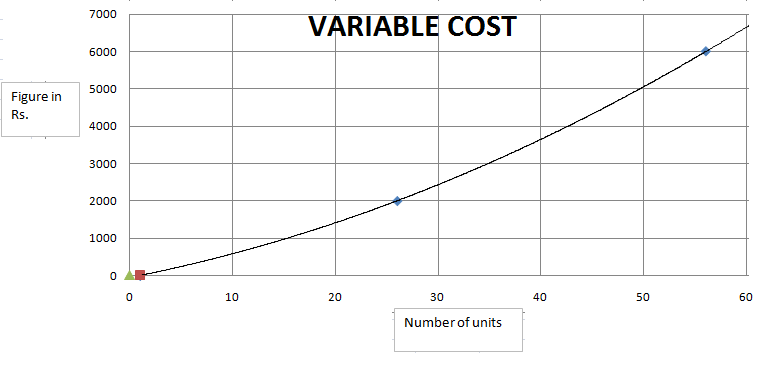### CAT 2000 Question Paper Question 37

Instructions

Answer these questions based on the following Information:

ABC Ltd. produces widgets for which the demand is unlimited and they can sell all of their production. The graph below describes the monthly variable costs incurred by the company as a function of the quantity produced. In addition, operating the plant for one shift results in a fixed monthly cost of Rs. 800. Fixed monthly costs for second shift operation are estimated at Rs. 1200. Each shift operation provides capacity for producing 30 widgets per month.
Note: Average unit cost, AC = Total monthly costs/monthly production, and Marginal cost MC is the rate of change in total cost for unit change in quantity produced.Question 37

# For monthly production level in the range of 30 to 60 Units,

Solution

When we take some value and check for the variation , we find that actual variation is opposite to that of option C.

Upto a certain level, AC is greater than MC and then AC is less than MC.

For producing 31 units, the average cost is 1200 + 800 + 2400 = Rs. 4400.
Average cost = 4400/31 = Rs.142 (Approx).

Let us calculate the marginal cost at this point.
As the number of units increases from 31 to 40, the variable cost increases from Rs.2400 to Rs.3700.
Marginal cost = (3700-2400)/10 = 1300/10 = Rs.130.

MC < AC for 31 units.

Let us calculate MC and AC for 60 units.

Average cost = (2000 + 6800)/60 = 8800/60 = Rs.146.66
As the number of units increases from 50 to 60, the variable cost increases from Rs.5000 to Rs.6800.
Marginal cost = Rs.1800/10 = Rs.180

MC > AC for 60 units.
As we can see, the trend is the inverse of what is mentioned in option C. Since none of the options capture the trend precisely, option D is the right answer.